# 概率图模型学习笔记：HMM、MEMM、CRF

### From Scofield. RxNLP

#### 二、Prerequisite

2.1 概率图
2.1.1 概览
2.1.2 有向图 vs. 无向图
2.1.3 马尔科夫假设&马尔科夫性
2.2 判别式模型 vs. 生成式模型
2.3 序列建模

#### 三、HMM

3.1 理解HMM
3.2 模型运行过程
3.2.1 学习过程
3.2.2 序列标注（解码）过程
3.2.3 序列概率过程

#### 四、MEMM

4.1 理解MEMM
4.2 模型运行过程
4.2.1 学习过程
4.2.2 序列标注过程
4.2.3 序列概率过程
4.3 标注偏置？

#### 五、CRF

5.1 理解CRF
5.2 模型运行过程
5.2.1 学习过程
5.2.2 序列标注过程
5.2.3 序列概率过程
5.3 CRF++分析
5.4 LSTM+CRF

# 一、Preface

a.训练模型参数，得到模型（由参数唯一确定），
b.预测给定的测试数据。

a.完整特征工程竞赛
b.野博客理论入门理解
c.再回到代码深入理解模型内部
d.再跨理论，查阅经典理论巨作。这时感性理性都有一定高度，会遇到很多很大的理解上的疑惑，这时3大经典可能就可以发挥到最大作用了。

# 二、Prerequisite

### 2.1 概率图

#### 2.1.1 概览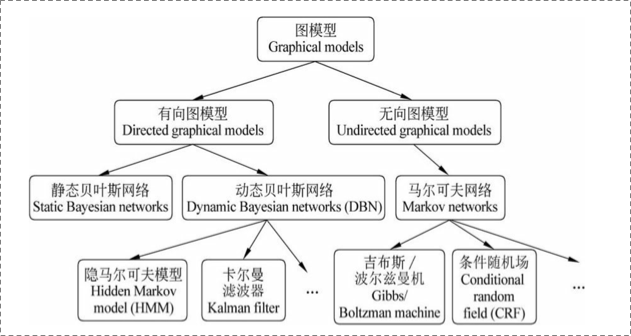• $V$$V$表示节点，即随机变量（放在此处的，可以是一个token或者一个label），具体地，用$Y=\left({y}_{1},\cdots ,{y}_{n}\right)$$Y = (y_{1}, {\cdots}, y_{n} )$为随机变量建模，注意$Y$$Y$现在是代表了一批随机变量（想象对应一条sequence，包含了很多的token），$P\left(Y\right)$$P(Y)$为这些随机变量的分布；
• $E$$E$表示边，即概率依赖关系。具体咋理解，还是要在后面结合HMM或CRF的graph具体解释。

#### 2.1.2 有向图 vs. 无向图

##### 1. 有向图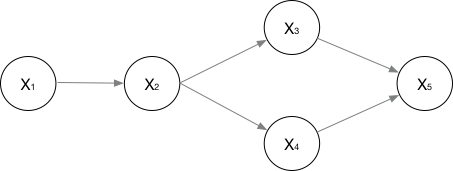$P\left({x}_{1},\cdots ,{x}_{n}\right)=P\left({x}_{1}\right)·P\left({x}_{2}|{x}_{1}\right)·P\left({x}_{3}|{x}_{2}\right)·P\left({x}_{4}|{x}_{2}\right)·P\left({x}_{5}|{x}_{3},{x}_{4}\right)$

##### 2. 无向图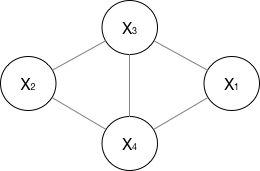$P\left(Y\right)=\frac{1}{Z\left(x\right)}\prod _{c}{\psi }_{c}\left({Y}_{c}\right)$

, 其中$Z\left(x\right)=\sum _{Y}\prod _{c}{\psi }_{c}\left({Y}_{c}\right)$$Z(x) = \sum_{Y} \prod_{c}\psi_{c}(Y_{c} )$，公式应该不难理解吧，归一化是为了让结果算作概率。

$P\left(Y\right)=\frac{1}{Z\left(x\right)}\left({\psi }_{1}\left({X}_{1},{X}_{3},{X}_{4}\right)·{\psi }_{2}\left({X}_{2},{X}_{3},{X}_{4}\right)\right)$

${\psi }_{c}\left({Y}_{c}\right)={e}^{-E\left({Y}_{c}\right)}={e}^{\sum _{k}{\lambda }_{k}{f}_{k}\left(c,y|c,x\right)}$

$P\left(Y\right)=\frac{1}{Z\left(x\right)}\prod _{c}{\psi }_{c}\left({Y}_{c}\right)=\frac{1}{Z\left(x\right)}\prod _{c}{e}^{\sum _{k}{\lambda }_{k}{f}_{k}\left(c,y|c,x\right)}=\frac{1}{Z\left(x\right)}{e}^{\sum _{c}\sum _{k}{\lambda }_{k}{f}_{k}\left({y}_{i},{y}_{i-1},x,i\right)}$

### 2.2 判别式（generative）模型 vs. 生成式(discriminative)模型

1. A批模型是这么工作的，他们直接将数据的Y（或者label），根据所提供的features，学习，最后画出了一个明显或者比较明显的边界（具体怎么做到的？通过复杂的函数映射，或者决策叠加等等mechanism），这一点线性LR、线性SVM应该很明显吧。

2. B批模型是这么工作的，他们先从训练样本数据中，将所有的数据的分布情况摸透，然后最终确定一个分布，来作为我的所有的输入数据的分布，并且他是一个联合分布$P\left(X,Y\right)$$P(X,Y)$(注意X包含所有的特征${x}_{i}$$x_{i}$，Y包含所有的label)。然后我来了新的样本数据（inference），好，我通过我学习来的模型的联合分布$P\left(X,Y\right)$$P(X,Y)$，再结合新样本给的X，通过条件概率就能出来Y：

$P\left(Y|X\right)=\frac{P\left(X,Y\right)}{P\left(X\right)}$

##### 1. 判别式模型

1. $P\left(Y|X\right)$$P(Y|X)$建模
2. 对所有的样本只构建一个模型，确认总体判别边界
3. 观测到输入什么特征，就预测最可能的label
4. 另外，判别式的优点是：对数据量要求没生成式的严格，速度也会快，小数据量下准确率也会好些。
##### 2. 生成式模型

1. $P\left(X,Y\right)$$P(X,Y)$建模
2. 这里我们主要讲分类问题，所以是要对每个label（${y}_{i}$$y_{i}$）都需要建模，最终选择最优概率的label为结果，所以没有什么判别边界。（对于序列标注问题，那只需要构件一个model）
3. 中间生成联合分布，并可生成采样数据。
4. 生成式模型的优点在于，所包含的信息非常齐全，我称之为“上帝信息”，所以不仅可以用来输入label，还可以干其他的事情。生成式模型关注结果是如何产生的。但是生成式模型需要非常充足的数据量以保证采样到了数据本来的面目，所以速度相比之下，慢。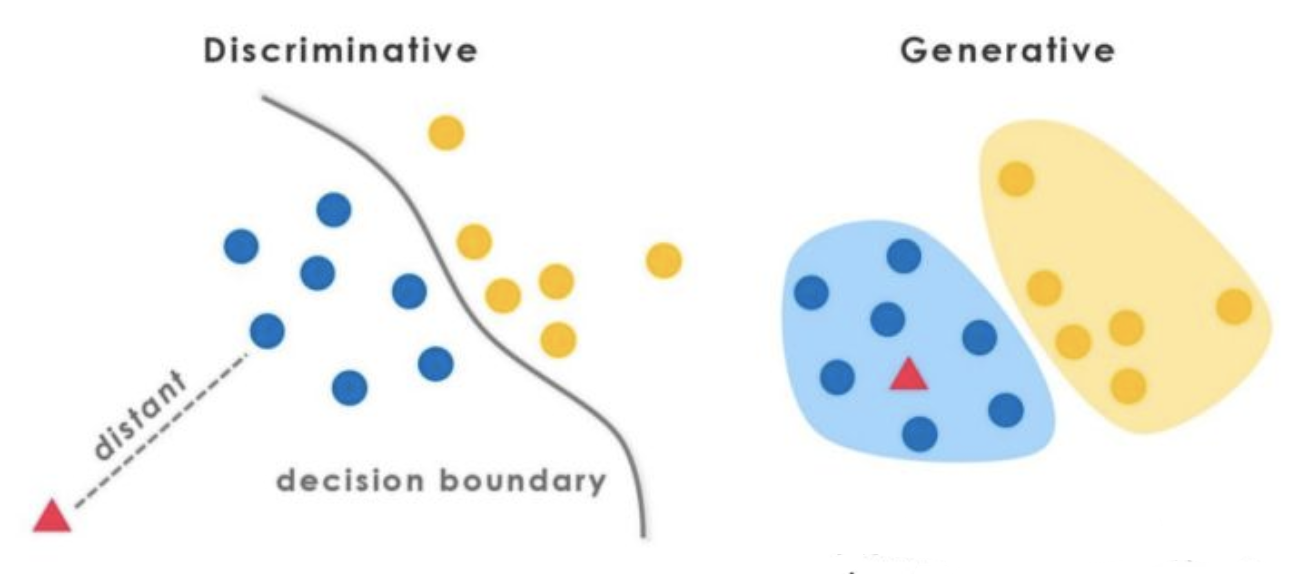### 2.3 序列建模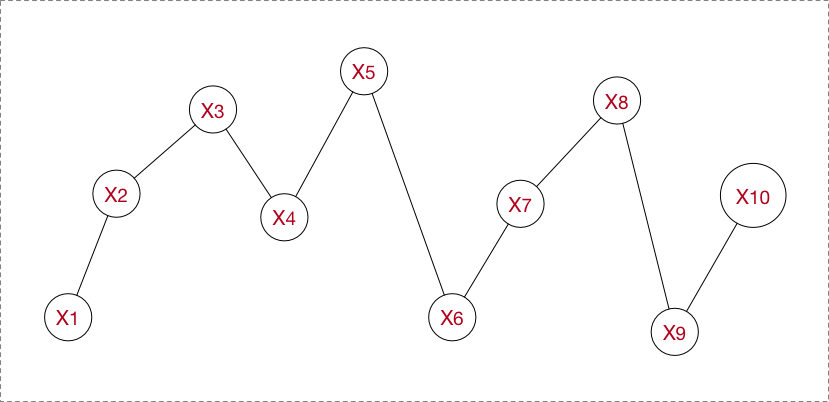• 节点之间有关联依赖性/无关联依赖性
• 序列的节点是随机的/确定的
• 序列是线性变化/非线性的
• ……

1. 拟合，预测未来节点（或走势分析）：
• 常规序列建模方法：AR、MA、ARMA、ARIMA
• 回归拟合
• Neural Networks
• 判断不同序列类别，即分类问题：HMM、CRF、General Classifier（ML models、NN models）
• 不同时序对应的状态的分析，即序列标注问题：HMM、CRF、RecurrentNNs

# 三、HMM

### 3.1 理解HMM

HMM属于典型的生成式模型。对照2.1的讲解，应该是要从训练数据中学到数据的各种分布，那么有哪些分布呢以及是什么呢？直接正面回答的话，正是HMM的5要素，其中有2个就是整个数据的不同角度的概率分布：

• $N$$N$，隐藏状态集$N=\left\{{q}_{1},\cdots ,{q}_{N}\right\}$$N = \lbrace q_{1}, \cdots, q_{N} \rbrace$, 我的隐藏节点不能随意取，只能限定取包含在隐藏状态集中的符号。
• $M$$M$，观测集$M=\left\{{v}_{1},\cdots ,{v}_{M}\right\}$$M = \lbrace v_{1}, \cdots, v_{M} \rbrace$, 同样我的观测节点不能随意取，只能限定取包含在观测状态集中的符号。
• $A$$A$，状态转移概率矩阵，这个就是其中一个概率分布。他是个矩阵，$A=\left[{a}_{ij}{\right]}_{N×N}$$A = [a_{ij}]_{N \times N}$（N为隐藏状态集元素个数），其中${a}_{ij}=P\left({i}_{t+1}|{i}_{t}\right)$$a_{ij} = P(i_{t+1}|i_{t})$,${i}_{t}$$i_{t}$即第i个隐状态节点,即所谓的状态转移嘛。
• $B$$B$，观测概率矩阵，这个就是另一个概率分布。他是个矩阵，$B=\left[{b}_{ij}{\right]}_{N×M}$$B = [b_{ij}]_{N \times M}$（N为隐藏状态集元素个数，M为观测集元素个数），其中${b}_{ij}=P\left({o}_{t}|{i}_{t}\right)$$b_{ij} = P(o_{t}|i_{t})$,${o}_{t}$$o_{t}$即第i个观测节点,${i}_{t}$$i_{t}$即第i个隐状态节点,即所谓的观测概率（发射概率）嘛。
• $\pi$$\pi$，在第一个隐状态节点${i}_{t}$$i_{t}$,我得人工单独赋予，我第一个隐状态节点的隐状态是$N$$N$中的每一个的概率分别是多少，然后$\pi$$\pi$就是其概率分布。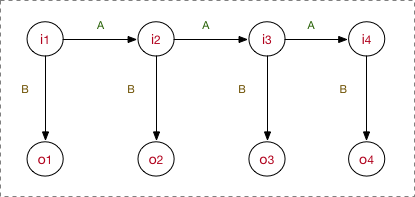input: “学习出一个模型，然后再预测出一条指定”
expected output: 学/B 习/E 出/S 一/B 个/E 模/B 型/E ，/S 然/B 后/E 再/E 预/B 测/E ……

1. 根据概率图分类，可以看到HMM属于有向图，并且是生成式模型，直接对联合概率分布建模$P\left(O,I\right)=\sum _{t=1}^{T}P\left({O}_{t}|{O}_{t-1}\right)P\left({I}_{t}|{O}_{t}\right)$$P(O,I) = \sum_{t=1}^{T}P(O_{t} | O_{t-1})P(I_{t} | O_{t})$(注意，这个公式不在模型运行的任何阶段能体现出来，只是我们都去这么来表示HMM是个生成式模型，他的联合概率$P\left(O,I\right)$$P(O,I)$就是这么计算的)。
2. 并且B中${b}_{ij}=P\left({o}_{t}|{i}_{t}\right)$$b_{ij} = P(o_{t}|i_{t})$，这意味着o对i有依赖性。
3. 在A中，${a}_{ij}=P\left({i}_{t+1}|{i}_{t}\right)$$a_{ij} = P(i_{t+1}|i_{t})$，也就是说只遵循了一阶马尔科夫假设，1-gram。试想，如果数据的依赖超过1-gram，那肯定HMM肯定是考虑不进去的。这一点限制了HMM的性能。

### 3.2 模型运行过程

#### 3.2.1 学习训练过程

• 极大似然估计, with 隐状态序列
• Baum-Welch(前向后向), without 隐状态序列

##### 1. 极大似然估计

• step1. 算A
$\stackrel{^}{{a}_{ij}}=\frac{{A}_{ij}}{\sum _{j=1}^{N}{A}_{ij}}$
• step2. 算B
$\stackrel{^}{{b}_{j}}\left(k\right)=\frac{{B}_{jk}}{\sum _{k=1}^{M}{B}_{jk}}$
• step3. 直接估计$\pi$$\pi$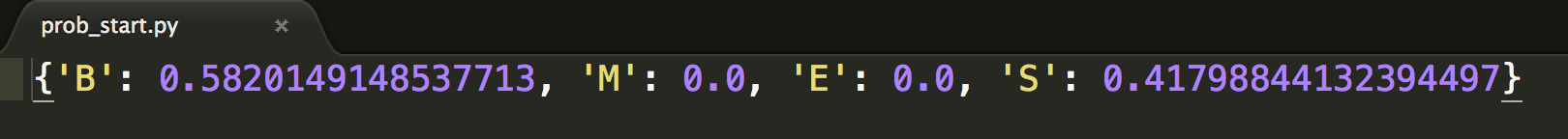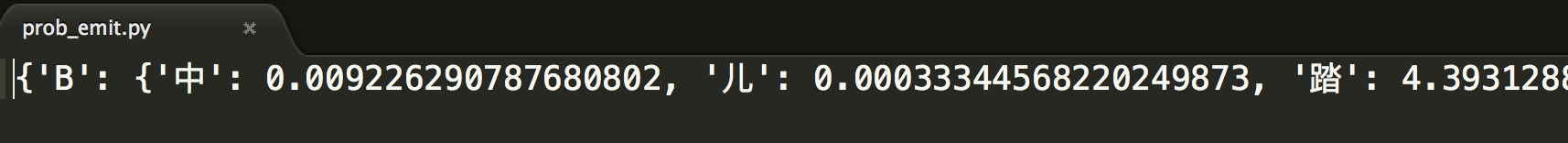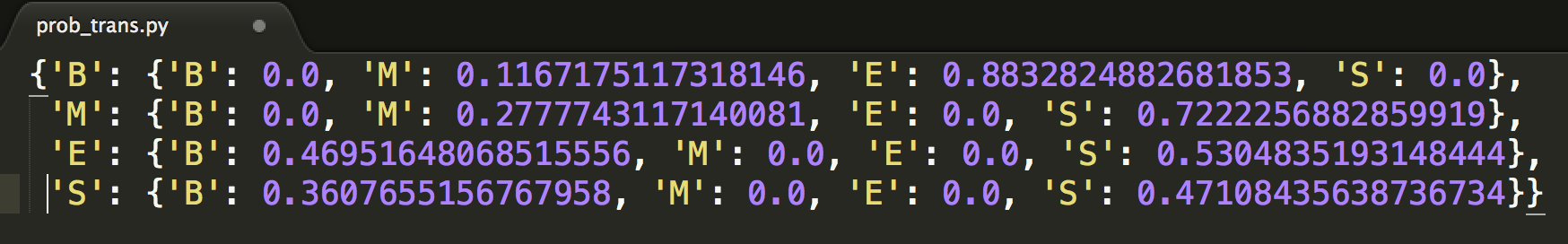#### 3.2.2 序列标注（解码）过程

${Q}_{max}=argma{x}_{allQ}\frac{P\left(Q,O\right)}{P\left(O\right)}$

Viterbi计算有向无环图的一条最大路径，应该还好理解。如图：#### 3.2.3 序列概率过程

HMM建模过程：

• 训练：我将所有A说的语音数据作为dataset_A,将所有B说的语音数据作为dataset_B（当然，先要分别对dataset A ,B做预处理encode为元数据节点，形成sequences）,然后分别用dataset_A、dataset_B去训练出HMM_A/HMM_B
• inference：来了一条新的sample（sequence），我不知道是A的还是B的，没问题，分别用HMM_A/HMM_B计算一遍序列的概率得到${P}_{A}（S）$$P_{A}（S）$${P}_{B}（S）$$P_{B}（S）$，比较两者大小，哪个概率大说明哪个更合理，更大概率作为目标类别。

• 直接计算法（穷举搜索）
• 前向算法
• 后向算法

# 四、MEMM

MEMM，即最大熵马尔科夫模型，这个是在接触了HMM、CRF之后才知道的一个模型。说到MEMM这一节时，得转换思维了，因为现在这MEMM属于判别式模型。

### 4.1 理解MEMM

HMM中，观测节点${o}_{i}$$o_{i}$依赖隐藏状态节点${i}_{i}$$i_{i}$,也就意味着我的观测节点只依赖当前时刻的隐藏状态。但在更多的实际场景下，观测序列是需要很多的特征来刻画的，比如说，我在做NER时，我的标注${i}_{i}$$i_{i}$不仅跟当前状态${o}_{i}$$o_{i}$相关，而且还跟前后标注${o}_{j}\left(j\ne i\right),$$o_{j}(j \neq i),$相关，比如字母大小写、词性等等。

$P\left(I|O\right)=\prod _{t=1}^{n}P\left({i}_{i}|{i}_{i-1},{o}_{i}\right),i=1,\cdots ,n$

$P\left(i|{i}^{{}^{\prime }},o\right)=\frac{1}{Z\left(o,{i}^{{}^{\prime }}\right)}exp\left(\sum _{a}\right){\lambda }_{a}{f}_{a}\left(o,i\right)$

$\begin{array}{}\text{(1)}& {f}_{a}\left(o,i\right)=\left\{\begin{array}{ll}1& \text{满足特定条件}，\\ 0& \text{other}\end{array}\end{array}$

$P\left(I|O\right)=\prod _{t=1}^{n}\frac{exp\left(\sum _{a}\right){\lambda }_{a}{f}_{a}\left(o,i\right)}{Z\left(o,{i}_{i-1}\right)},i=1,\cdots ,n$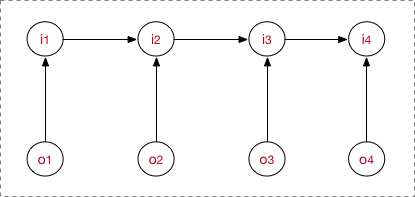MEMM需要两点注意：

1. 与HMM的${o}_{i}$$o_{i}$依赖${i}_{i}$$i_{i}$不一样，MEMM当前隐藏状态${i}_{i}$$i_{i}$应该是依赖当前时刻的观测节点${o}_{i}$$o_{i}$和上一时刻的隐藏节点${i}_{i-1}$$i_{i-1}$
2. 需要注意，之所以图的箭头这么画，是由MEMM的公式决定的，而公式是creator定义出来的。

step1. 先预定义特征函数${f}_{a}\left(o,i\right)$$f_{a}(o,i)$
step2. 在给定的数据上，训练模型，确定参数，即确定了MEMM模型
step3. 用确定的模型做序列标注问题或者序列求概率问题。

### 4.2 模型运行过程

MEMM模型的工作流程也包括了学习训练问题、序列标注问题、序列求概率问题。

### 4.3 标注偏置？

MEMM讨论的最多的是他的labeling bias 问题。

#### 1. 现象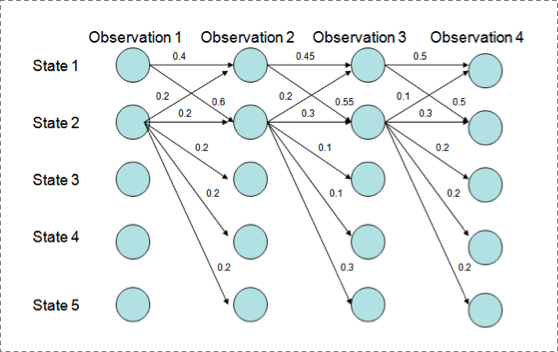P(1-> 1-> 1-> 1)= 0.4 x 0.45 x 0.5 = 0.09 ，
P(2->2->2->2)= 0.2 X 0.3 X 0.3 = 0.018，
P(1->2->1->2)= 0.6 X 0.2 X 0.5 = 0.06，
P(1->1->2->2)= 0.4 X 0.55 X 0.3 = 0.066

#### 2. 解释原因

$P\left(I|O\right)=\prod _{t=1}^{n}\frac{exp\left(\sum _{a}\right){\lambda }_{a}{f}_{a}\left(o,i\right)}{Z\left(o,{i}_{i-1}\right)},i=1,\cdots ,n$

$\sum$$\sum$求和的作用在概率中是归一化，但是这里归一化放在了指数内部，管这叫local归一化。

${\delta }_{i+1}=ma{x}_{1\le j\le m}\left\{{\delta }_{i}\left(I\right)+\sum _{i}^{T}\sum _{k}^{M}{\lambda }_{k}{f}_{k}\left(O,{I}_{i-1},{I}_{i},i\right)\right\}$

# 五、CRF

### 5.1 理解CRF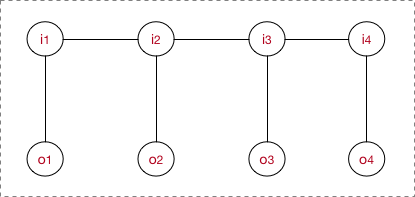$P\left(Y|X\right)=\frac{1}{Z\left(x\right)}\prod _{c}{\psi }_{c}\left({Y}_{c}|X\right)=\frac{1}{Z\left(x\right)}\prod _{c}{e}^{\sum _{k}{\lambda }_{k}{f}_{k}\left(c,y|c,x\right)}=\frac{1}{Z\left(x\right)}{e}^{\sum _{c}\sum _{k}{\lambda }_{k}{f}_{k}\left({y}_{i},{y}_{i-1},x,i\right)}$

$P\left(I|O\right)=\frac{1}{Z\left(O\right)}\prod _{i}{\psi }_{i}\left({I}_{i}|O\right)=\frac{1}{Z\left(O\right)}\prod _{i}{e}^{\sum _{k}{\lambda }_{k}{f}_{k}\left(O,{I}_{i-1},{I}_{i},i\right)}=\frac{1}{Z\left(O\right)}{e}^{\sum _{i}\sum _{k}{\lambda }_{k}{f}_{k}\left(O,{I}_{i-1},{I}_{i},i\right)}$

#### 1. 特征函数

$P\left(I|O\right)=\frac{1}{Z\left(O\right)}{e}^{\sum _{i}^{T}\sum _{k}^{M}{\lambda }_{k}{f}_{k}\left(O,{I}_{i-1},{I}_{i},i\right)}$

• 下标i表示我当前所在的节点（token）位置。
• 下标k表示我这是第几个特征函数，并且每个特征函数都附属一个权重${\lambda }_{k}$$\lambda_{k}$，也就是这么回事，每个团里面，我将为$toke{n}_{i}$$token_{i}$构造M个特征，每个特征执行一定的限定作用，然后建模时我再为每个特征函数加权求和。
• $Z\left(O\right)$$Z(O)$是用来归一化的，为什么？想想LR以及softmax为何有归一化呢，一样的嘛，形成概率值。
• 再来个重要的理解。$P\left(I|O\right)$$P(I | O)$这个表示什么？具体地，表示了在给定的一条观测序列$O=\left({o}_{1},\cdots ,{o}_{i}\right)$$O=(o_{1},\cdots, o_{i})$条件下，我用CRF所求出来的隐状态序列$I=\left({i}_{1},\cdots ,{i}_{i}\right)$$I=(i_{1},\cdots, i_{i})$的概率，注意，这里的I是一条序列，有多个元素（一组随机变量），而至于观测序列$O=\left({o}_{1},\cdots ,{o}_{i}\right)$$O=(o_{1},\cdots, o_{i})$，它可以是一整个训练语料的所有的观测序列；也可以是在inference阶段的一句sample，比如说对于序列标注问题，我对一条sample进行预测，可能能得到${P}_{j}\left(I|O\right)（j=1,\dots ,J）$$P_{j}(I | O)（j=1,…,J）$J条隐状态I，但我肯定最终选的是最优概率的那条（by viterbi）。这一点希望你能理解。

$P\left(I|O\right)=\frac{1}{Z\left(O\right)}{e}^{\sum _{i}^{T}\sum _{k}^{M}{\lambda }_{k}{f}_{k}\left(O,{I}_{i-1},{I}_{i},i\right)}=\frac{1}{Z\left(O\right)}{e}^{\left[\sum _{i}^{T}\sum _{j}^{J}{\lambda }_{j}{t}_{j}\left(O,{I}_{i-1},{I}_{i},i\right)+\sum _{i}^{T}\sum _{l}^{L}{\mu }_{l}{s}_{l}\left(O,{I}_{i},i\right)\right]}$

• ${t}_{j}\mathrm{}为i处的转移特征，对应权重$$t_{j}\为i处的转移特征，对应权重$\lambda_{j}$,每个$$,每个$token_{i}$都有J个特征.转移特征针对的是前后token之间的限定。 • 举个例子： $\begin{array}{}\text{(2)}& {t}_{k=1}\left(o,i\right)=\left\{\begin{array}{ll}1& \text{满足特定转移条件，比如前一个token是‘I’}，\\ 0& \text{other}\end{array}\end{array}$ • ${s}_{l}\mathrm{}为i处的状态特征，对应权重$$s_{l}\为i处的状态特征，对应权重$\mu_{l}$,每个$$,每个$token_{i}$都有L个特征
• 举个例子：
$\begin{array}{}\text{(3)}& {s}_{l=1}\left(o,i\right)=\left\{\begin{array}{ll}1& \text{满足特定状态条件，比如当前token的POS是‘V’}，\\ 0& \text{other}\end{array}\end{array}$

$P\left(I|O\right)=\frac{1}{Z\left(O\right)}{e}^{\sum _{i}^{T}\sum _{k}^{M}{\lambda }_{k}{f}_{k}\left(O,{I}_{i-1},{I}_{i},i\right)}$

$Score=\sum _{i}^{T}\sum _{k}^{M}{\lambda }_{k}{f}_{k}\left(O,{I}_{i-1},{I}_{i},i\right)$

log-linear models take the following form:

$P\left(y|x;\omega \right)=\frac{exp\left(\omega ·\varphi \left(x,y\right)\right)}{\sum _{{y}^{{}^{\prime }}\in Y}exp\left(\omega ·\varphi \left(x,{y}^{{}^{\prime }}\right)\right)}$

### 5.2 模型运行过程

• step1. 先预定义特征函数${f}_{a}\left(o,i\right)$$f_{a}(o,i)$
• step2. 在给定的数据上，训练模型，确定参数${\lambda }_{k}$$\lambda_{k}$
• step3. 用确定的模型做序列标注问题或者序列求概率问题

……

#### 5.2.2 序列标注过程

${\delta }_{i+1}=ma{x}_{1\le j\le m}\left\{{\delta }_{i}\left(I\right)+\sum _{i}^{T}\sum _{k}^{M}{\lambda }_{k}{f}_{k}\left(O,{I}_{i-1},{I}_{i},i\right)\right\}$

### 5.3 CRF++分析

Nuclear
theory
devoted
major
efforts
……

B_MAT
B_PRO
B_TAS
E_MAT
E_PRO
E_TAS
I_MAT
I_PRO
I_TAS
O
S_MAT
S_PRO
S_TAS

#### 1. 定义模板

CRF++的模板（template）有U系列（unigram）、B系列(bigram)，不过我至今搞不清楚B系列的作用，因为U模板都可以完成2-gram的作用。

U00:%x[-2,0]
U01:%x[-1,0]
U02:%x[0,0]
U03:%x[1,0]
U04:%x[2,0]

U05:%x[-2,0]/%x[-1,0]/%x[0,0]
U06:%x[-1,0]/%x[0,0]/%x[1,0]
U07:%x[0,0]/%x[1,0]/%x[2,0]
U08:%x[-1,0]/%x[0,0]
U09:%x[0,0]/%x[1,0]

B

#### 2. 产生特征函数

U00 - U04的模板产生的是状态特征函数；U05 - U09的模板产生的是转移特征函数。

22607 B
790309 U00:%
3453892 U00:%)
2717325 U00:&
2128269 U00:’t
2826239 U00:(0.3534
2525055 U00:(0.593–1.118
197093 U00:(1)
2079519 U00:(1)L=14w2−12w−FμνaFaμν
2458547 U00:(1)δn=∫−∞En+1ρ˜(E)dE−n
1766024 U00:(1.0g
2679261 U00:(1.1wt%)
1622517 U00:(100)
727701 U00:(1000–5000A)
2626520 U00:(10a)
2626689 U00:(10b)
……
2842814 U07:layer/thicknesses/Using
2847533 U07:layer/thicknesses/are
2848651 U07:layer/thicknesses/in
331539 U07:layer/to/the
1885871 U07:layer/was/deposited
……（数量非常庞大）

func1 = if (output = B and feature=”U02:一”) return 1 else return 0
func2 = if (output = M and feature=”U02:一”) return 1 else return 0
func3 = if (output = E and feature=”U02:一”) return 1 else return 0
func4 = if (output = S and feature=”U02:一”) return 1 else return 0

#### 3. 求参

0.3972716048310705
0.5078838237171732
0.6715316559507898
-0.4198827647512405
-0.4233310655891150
-0.4176580083832543
-0.4860489836004728
-0.6156475863742051
-0.6997919485753300
0.8309956709647820
0.3749695682658566
0.2627347894057647
0.0169732441379157
0.3972716048310705
0.5078838237171732
0.6715316559507898
……（数量非常庞大，与每个label的特征函数对应，我这有300W个）

Nuclear B_TAS
theory E_TAS
devoted O
major O
efforts O
……

### 5.4 LSTM+CRF

LSTM+CRF这个组合其实我在知乎上答过问题，然后顺便可以整合到这里来。

#### 2、LSTM+CRF

input: “学习出一个模型，然后再预测出一条指定”

expected output: 学/B 习/E 出/S 一/B 个/E 模/B 型/E ，/S 然/B 后/E 再/E 预/B 测/E ……

real output: 学/B 习/E 出/S 一/B 个/B 模/B 型/E ，/S 然/B 后/B 再/E 预/B 测/E ……

LSTM+CRF codes, here. Go just take it.

# 六、总结

### 1. 总体对比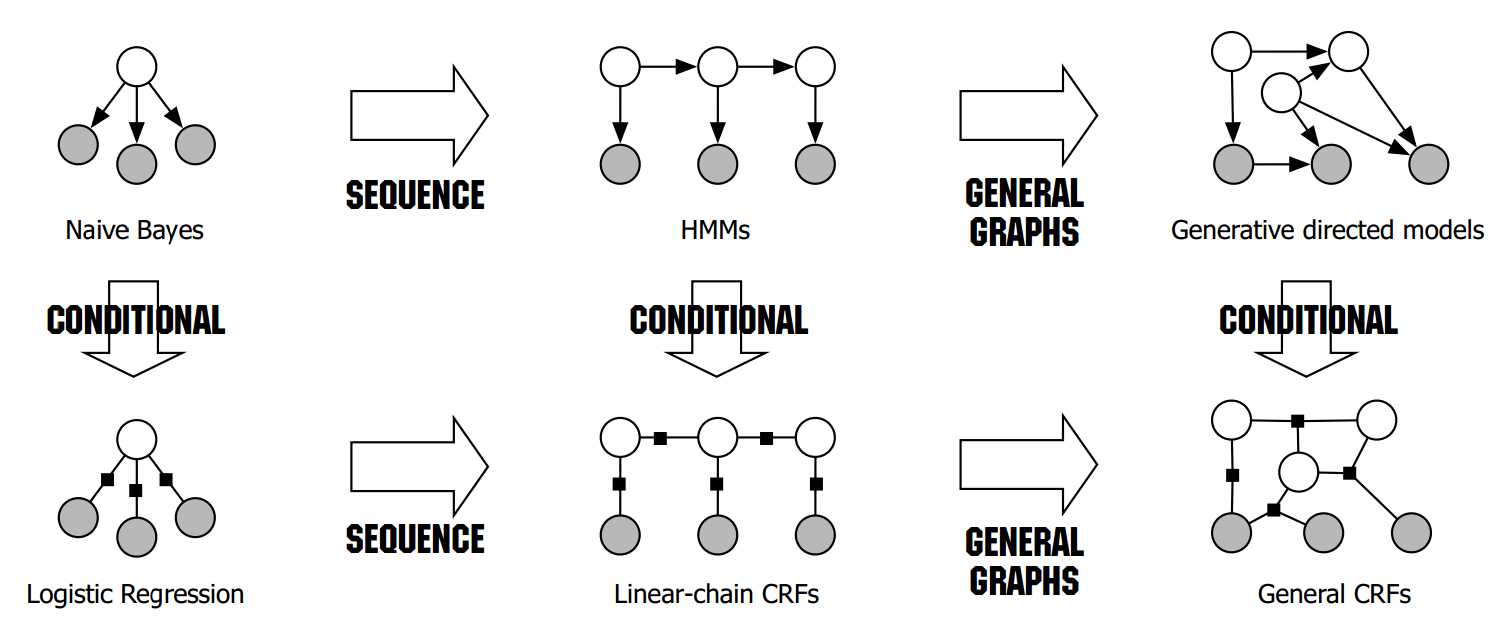### 2. HMM vs. MEMM vs. CRF

1. HMM -> MEMM：
HMM模型中存在两个假设：一是输出观察值之间严格独立，二是状态的转移过程中当前状态只与前一状态有关。但实际上序列标注问题不仅和单个词相关，而且和观察序列的长度，单词的上下文，等等相关。MEMM解决了HMM输出独立性假设的问题。因为HMM只限定在了观测与状态之间的依赖，而MEMM引入自定义特征函数，不仅可以表达观测之间的依赖，还可表示当前观测与前后多个状态之间的复杂依赖。
2. MEMM -> CRF:
• CRF不仅解决了HMM输出独立性假设的问题，还解决了MEMM的标注偏置问题，MEMM容易陷入局部最优是因为只在局部做归一化，而CRF统计了全局概率，在做归一化时考虑了数据在全局的分布，而不是仅仅在局部归一化，这样就解决了MEMM中的标记偏置的问题。使得序列标注的解码变得最优解。
• HMM、MEMM属于有向图，所以考虑了x与y的影响，但没讲x当做整体考虑进去（这点问题应该只有HMM）。CRF属于无向图，没有这种依赖性，克服此问题。

# Referrences:

《统计学习方法》，李航
《统计自然语言处理》，宗成庆
《 An Introduction to Conditional Random Fields for Relational Learning》， Charles Sutton， Andrew McCallum
《Log-Linear Models, MEMMs, and CRFs》，ichael Collins©️2019 CSDN 皮肤主题: 编程工作室 设计师: CSDN官方博客